1年前   阅读数 145 0

# 什么是C语言

C语言的定义百度不难获取。C语言是一门通用计算机编程语言，广泛应用于底层开发。那什么是计算机编程语言和底层开发呢？

## 什么是底层开发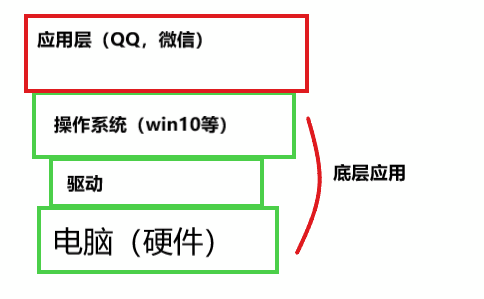## ASCII码表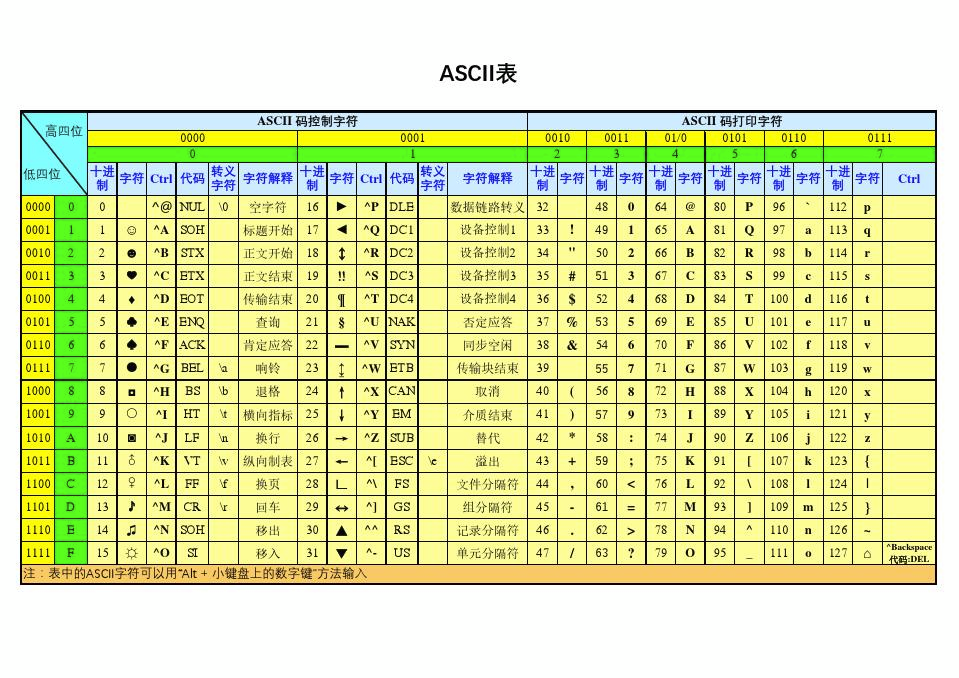# 第一个C语言程序

## 创建工程 以VS2019为例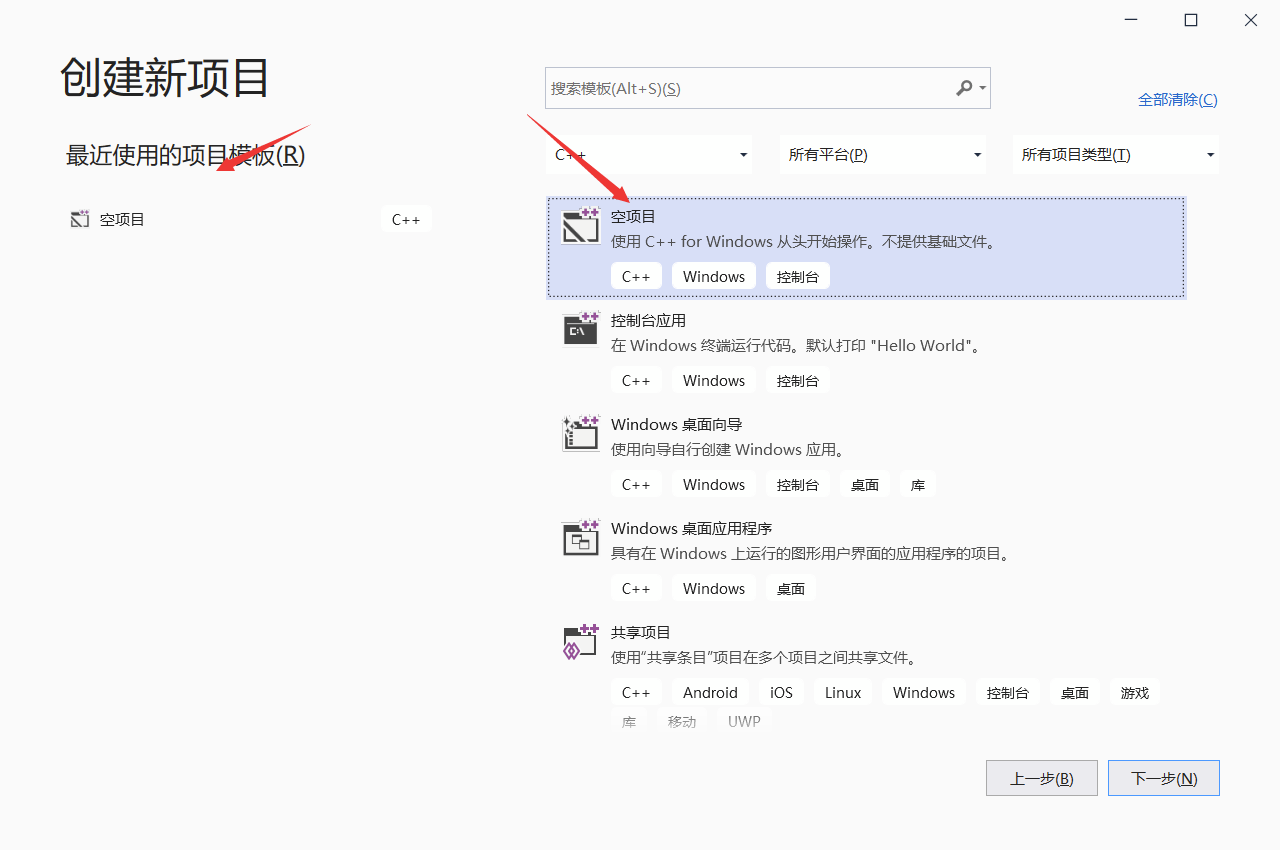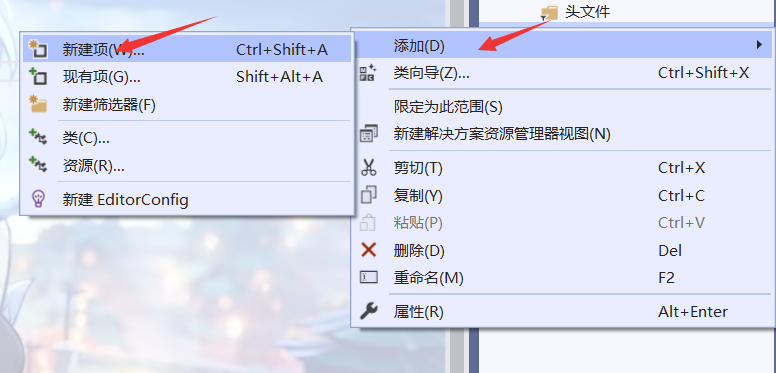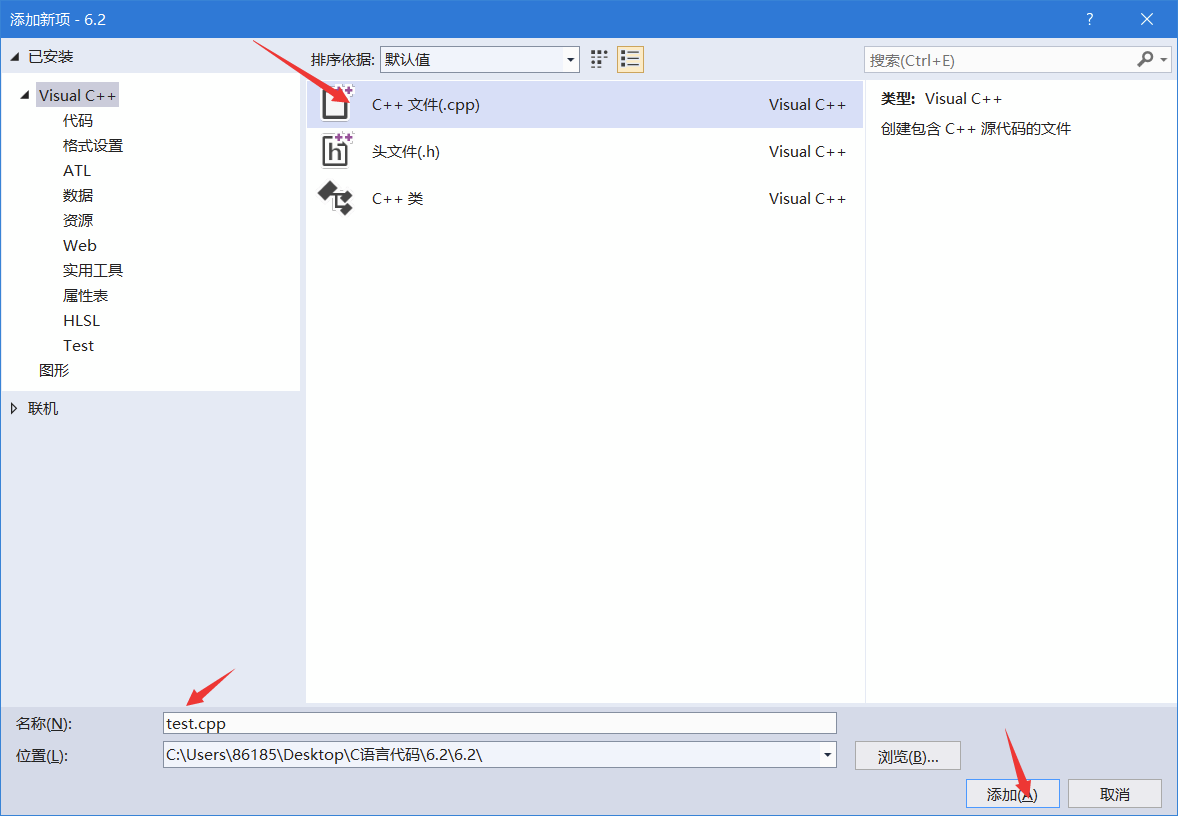这样我们便创建了一个新的C语言文件，接下来就可以写代码了。

## 主函数

``````int main()
{

return 0;
}
``````

int是函数返回值的类型，最常用的是整型
main是主函数的函数名
（）是函数的参数，由于主函数一般情况下为无参，因此（）里面的内容可以为空，
{}里面是主函数的内容
return 0;表示的是函数的返回值

``````#include<stdio.h>
int main()
{

printf("hello world");
return 0;
}
``````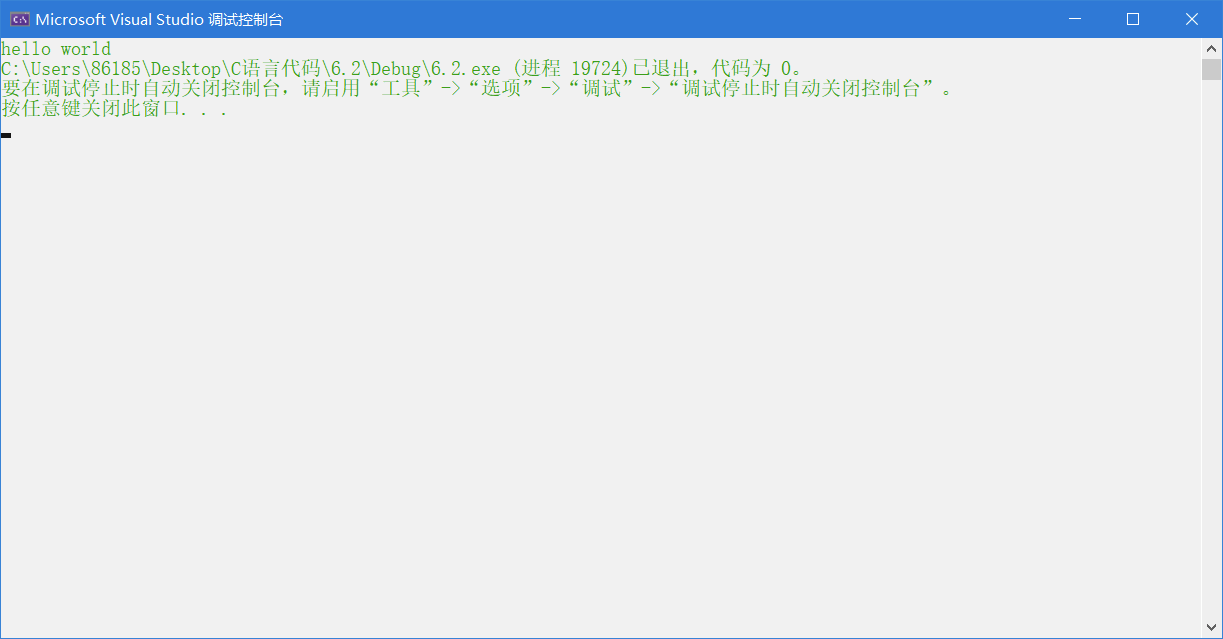如此一来，我们的第一个C语言程序就写好了。

# 数据类型

``````char      //字符数据类型,比如a、b、c、！等字符
short      //短整型 用来描述范围更小的整数
int         //整形 用来描述整数
long        //长整型 用来描述范围更大的整数
long long   //更长的整形 用来描述范围更大的整数
float       //单精度浮点数 用来描述小数
double      //双精度浮点数 比float精度更高
``````

## 不同数据类型的大小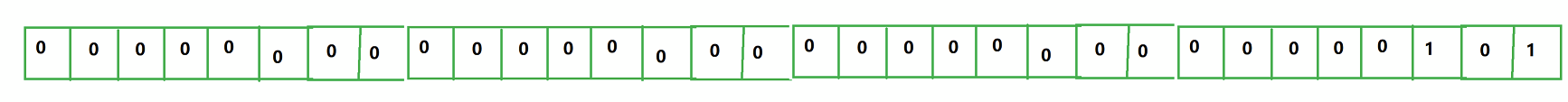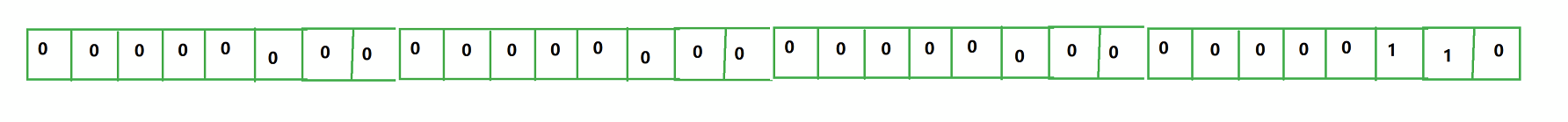以上就是比特和字节的概念。

1字节(Byte)=8位(bit)
1KB( Kilobyte，千字节)=1024B
1MB( Megabyte，兆字节)=1024KB
1GB( Gigabyte，吉字节，千兆)=1024MB
1TB( Trillionbyte，万亿字节，太字节)=1024GB
1PB( Petabyte，千万亿字节，拍字节)=1024TB
1EB( Exabyte，百亿亿字节，艾字节)=1024PB
1ZB(Zettabyte，十万亿亿字节，泽字节)=1024EB
1YB( Yottabyte，一亿亿亿字节，尧字节)=1024ZB
1BB( Brontobyte，千亿亿亿字节)=1024YB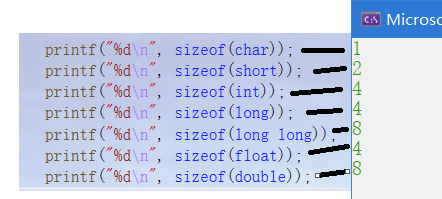## 数据类型的输出格式

``````    %d-打印整形
%c-打印字符
%f-打印浮点型-小数
%p-以地址形式打印
%x-打印16进制数字
%s-打印字符串
``````

``````int main()
{

int a=32;
printf("%x",a);//结果为20
return 0;
}
``````

%x是16进制输出，因此在打印输出时，会将a转换为16进制再进行输出。

# 变量、常量

## 定义变量的方法

``````int age=10;//age值为10，但由于age是变量，因此age的值可以被重新赋值
age=11;//此时age的值变为11
``````

``````float weight=45.5;
char ch='w';
``````

``````int age=10;

int age;
age=10
``````

## 局部变量和全局变量

``````int g=100;//全局变量

void test()//定义一个函数，以后会具体说明
{

int b=100;//局部变量
}

int main()
{

int a=10;//局部变量

return 0;
}
``````

``````int a=100;//全局变量
int main()
{

int a=10;//局部变量
printf("%d",a);// 局部变量优先，结果为10
return 0;
}
``````

### 变量的作用域和生命周期

#### 作用域

``````int b=20;
int main()
{

int c=30;

{

int a=10;
}
printf("a=%d",a);//打印会报错 无法打印输出
printf("b=%d",b);//可以打印输出

{

printf("c=%d",c);//可以打印输出
}
return 0;
}
``````

a是局部变量，a的定义范围是a所在的那个{}，它的作用域就是它所在的{}，出了{}以后a就不能使用了。
b是全局变量，作用域是整个工程，因此b的作用域并不受{}的限制。
c的是局部变量，c的作用域是整个main函数所在的范围，由于 printf(“c=%d”,c);所在的{}也属于main函数所在的范围，因此可以打印输出。

#### 生命周期

1. 局部变量的生命周期是：进入作用域生命周期开始，出作用域生命周期结束。
2. 全局变量的生命周期是：整个程序的生命周期，即从程序开始到程序结束。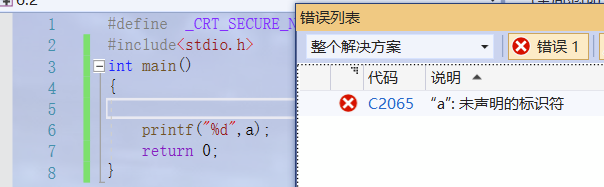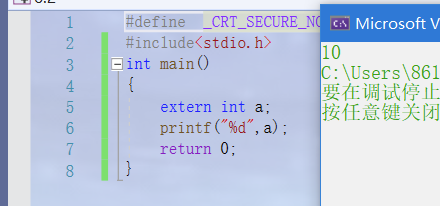## 常量

C语言中常量分为以下几种：

• 字面常量
程序中出现的数字，字符等。
``````#include<stdio.h>
int main()
{

int a = 10;
char b = 'w';

return 0;
}
``````

10和w就是字面常量。

• const 修饰的常变量（无法修改的变量称为常变量）
``````int main()
{

const int a = 10;

return 0;
}
``````

const修饰的常变量无法修改，比如下面的程序

``````#include<stdio.h>
int main()
{

const int a = 10;
a = 20;

return 0;
}
``````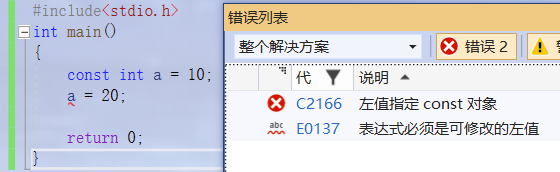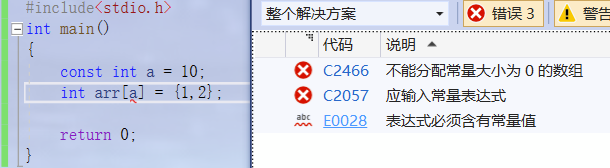• #define 定义的标识符常量

``````#include<stdio.h>
#define a 10
int main()
{

int arr[a] = {
0 };//相当于int arr = { 0 };
a=20;//无法修改，因为a具有字面常量的属性；
return 0;
}
``````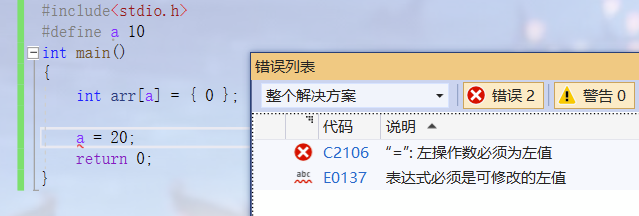• 枚举常量

# 字符串+转义字符+注释

## 字符串

C语言中由双引号引起的一串字符叫做字符串。

\0对于字符串非常重要，比如下面的代码：

``````int main()
{

char arr1[] = "abc";
char arr2[] = {
'a', 'b', 'c'};
char arr3[] = {
'a', 'b', 'c'， '\0'};
printf("%s\n", arr1);//abc
printf("%s\n", arr2);//abc和乱码
printf("%s\n", arr3);//abc
return 0;
}
``````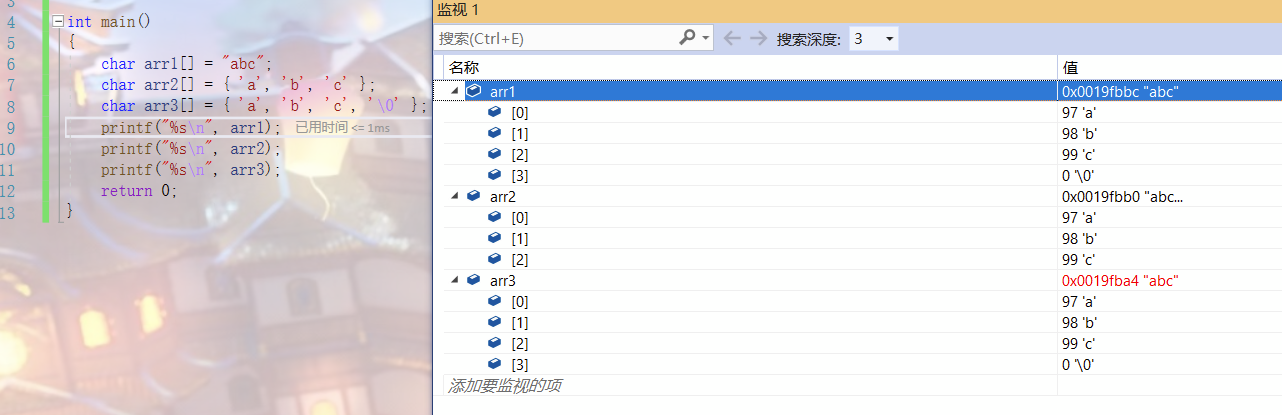arr1字符串后面有一个隐藏的\0 ，因此在打印输出时，遇到\0后，打印停止。
arr2字符串后面没有\0，因此在打印完abc以后还会继续打印，直到遇到\0才会停止。
arr3字符串有\0，遇到\0后，打印停止。

## 转义字符

\? 在书写连续多个问号时使用，防止他们被解析成三字母词
\ ’ 用于表示字符常量’
\“ 用于表示一个字符串内部的双引号
\\ 用于表示一个反斜杠，防止它被解释为一个转义序列符。
\a 警告字符，蜂鸣
\b 退格符
\f 进纸符
\n 换行
\r 回车
\t 水平制表符
\v 垂直制表符
\ddd ddd表示1~3个八进制的数字
\xdd dd表示2个十六进制数字

``````#include <stdio.h>
#include<string.h>
int main()
{

// \32被解析成一个转义字符
//\32 32是2个8进制数字
//32作为8进制代表的十进制数字的ASCII码值对应的字符
//100先转化为10进制为64，然后将64转化为ASCII码值代表的字符
printf("%d\n", strlen("c:\test\100\test.c"));//结果为13
return 0;
}
``````

strlen这个函数可以求字符串的长度，在这里\t和\100都是一个字符，因此一共有13个字符，输出结果为13。\t是水平制表符算作一个字符可以理解，那为什么\100也是一个字符呢？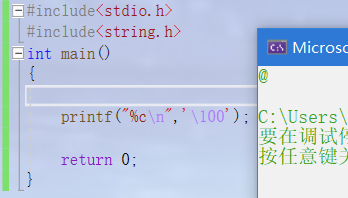### 补齐字符

``````int main()
{

int a = 123;
int b = 1;
printf("%3d\n%3d\n", a, b);//%3d是右对齐，如果输出的位数不够三位，就会补空格
printf("%3d\n%-3d\n", a, b);//%-3d是左对齐
}
``````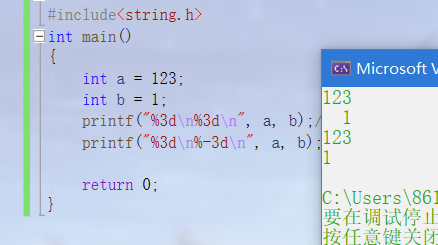## 注释

1. 代码中有不需要的代码可以直接删除，也可以注释掉
2. 代码中有些代码比较难懂，可以加一下注释文字
比如：
``````#include <stdio.h>
{

return x+y; }
/* int Sub(int x, int y) { return x-y; } */
int main()
{

//int a = 10;
return 0;
}
``````

• C语言风格的注释 /xxxxxx/
• 缺陷：不能嵌套注释
• C++风格的注释 //xxxxxxxx
• 可以注释一行也可以注释多行

# 选择语句

``````#include <stdio.h>
int main()
{

int coding = 0;
printf("1+1=？>");
scanf("%d", &coding);
if(coding == 2)
{

prinf("回答正确\n");
}
else
{

printf("回答错误\n");
}
return 0;
}
``````

# 循环语句

C语言中实现循环的方式：

• while语句
• for语句
• do … while语句

``````//while循环的实例
#include <stdio.h>
int main()
{

int line = 0;
while(line<20000)
{

line++;
printf("hello world\n");
}
return 0;
}
``````

# 函数

``````#include <stdio.h>
{

int z = x+y;
return z;
}
int main()
{

int num1 = 0;
int num2 = 0;
int sum = 0;
printf("输入两个操作数:>");
scanf("%d %d", &num1, &num2);
printf("sum = %d\n", sum);
return 0;
}
``````

# 数组

## 数组的定义

``````int arr = {
1,2,3,4,5,6,7,8,9,10};//定义一个整形数组，最多放10个整形元素
``````

int 是数组的类型；arr是数组的名字；是数组的大小，数组的大小也可以不写，编译器会自动识别数组的大小；{ }内是数组的每个元素。

## 数组的使用

``````#include <stdio.h>
int main()
{

int i = 0;
int arr = {
1,2,3,4,5,6,7,8,9,10};
for(i=0; i<10; i++)
{

printf("%d ", arr[i]);//用循环的方式打印数组中的每个元素
}
printf("\n");
return 0;
}
``````

# 操作符

## 算数操作符

``````+ - * / %   加 减 乘 除 取模(即求一个数除以另一个所得到的余数)
``````

## 移位操作符

``````>> <<    左移和右移
``````
• 位移操作符移动的是变量的二进制位而不是十进制位

``````int main()
{

int a = 1;
int b = a << 1;
printf("%d", b);

return 0;
}
``````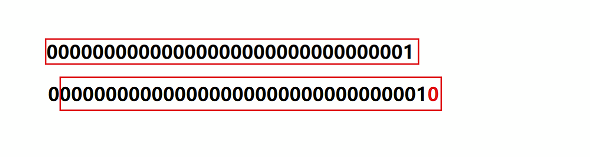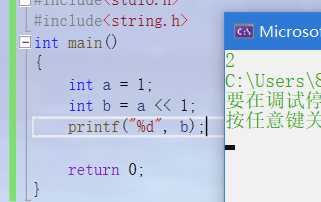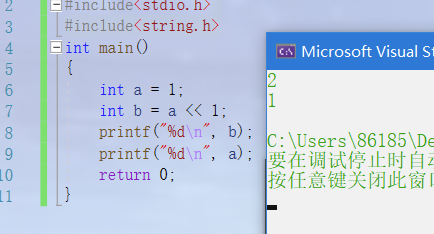a还是原来的a，本身的值并没有改变

## 位操作符

``````&  |  ^   与运算  或运算  异或运算
``````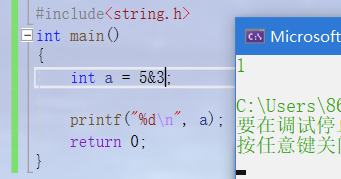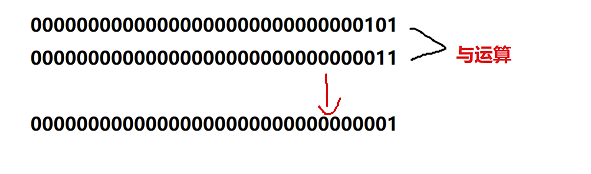## 赋值操作符

``````=   +=   -=    *=   /=    &=    ^=    |=      >>=      <<=
``````

= 将右边的值赋值给左边
+=对原本的操作进行了简化， a+=1 等价于 a=a+1 只是a+=1更简便一些， 其他操作符也是同理

## 单目操作符

``````!           逻辑反操作（将真变为假，假变为真，非0的数都是真，0为假）
-           负值
+           正值
&           取地址（与指针配合使用）
sizeof      操作数的类型长度（求数组的大小，单位是字节）
~           对一个数的二进制按位取反
--          前置、后置--
++          前置、后置++
*           间接访问操作符(解引用操作符)
(类型)       强制类型转换，将一个类型强制转换成另一个类型
``````

++在前，先自加1，再使用
++在后，先使用，再自加1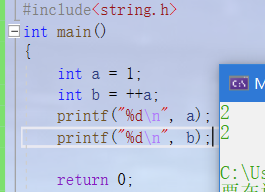a先自加1，再将自己的值赋值给b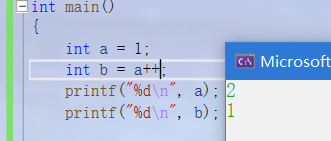a先将自己的值赋值给b，再自加1

## 关系操作符

``````>    //大于
>=    //大于等于
<     //小于
<=     //小于等于
!=    // “不相等”
==    //“相等”
``````

## 逻辑操作符

``````&&        逻辑与   所有表达式都为真（非0），那整体就为真（1），否则为假（0）
||        逻辑或   所有表达式有一个为真（非0），那么整体就为真（1），只有所有表达式都为假时整体才为假（0）
``````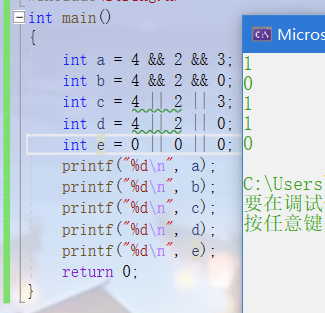## 条件操作符(三目运算符）

``````exp1 ? exp2 : exp3    如果表达式1的值为真，整体的值就是表达式2，如果表达式1的值为假，整体的值就是表达式3
``````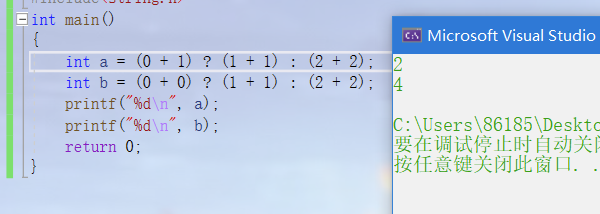## 逗号表达式

``````exp1, exp2, exp3, …expN  每个表达式从左向右依次进行计算，

``````
``````#include<stdio.h>
int main()
{

int a = 1;
int b = 3;
a = (b = a + 1, a = b + 1,b= a + b);
//先计算a+1的值然后将其赋值给b，此时b的值为2，a的值为1；
//再计算b+1的值然后将其赋值给a，此时a的值为3，b的值为2；
//最后计算a+b的值并将结果赋值给b，此时b的值为5
//整体表达式的结果就是最后一个表达式的结果，最后一个表达式的结果为5，因此整体表达式结果为5
printf("%d", a);
return 0;
}
``````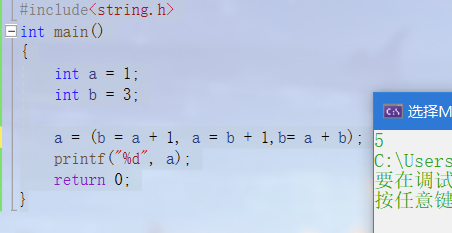## 下标引用、函数调用和结构成员

``````[]    用来访问数组中的成员，如arr就是访问数组的第二个成员（数组的下标从0开始）
()    用来调用函数时给函数传参
.     用来访问结构体成员
->   用来通过指针访问结构体成员

``````

# 常见关键字

C语言内置的关键字。只需初步了解即可。另外记住，变量名不能和关键字相同，要不然编译器无法区分关键字和变量名。

``````auto--自动  局部变量都是自动变量 但在编译器auto省略
break--循环中停止循环 switch选择语句中结束语句
case--switch语句中进行选择
char
const--常变量
continue--继续，运用在循环中
default--默认，用在switch选择中使用
do
double
else  --条件语句中使用
enum --枚举
extern--引入外部负号
float--单精度浮点数
for
goto
if --条件语句中使用
int
long
register--寄存器关键字，建议将变量定义为寄存器变量
return
short
signed--有符号数，
signed int 与 int 等价
sizeof
static--静态的 1.修饰局部变量，生命周期变长-静态局部变量 2.修饰全局变量，改变变量的作用域，使变量只能作用在自己所在的源文件使用，出了源文件将无法使用-静态全局变量 3.修饰函数，改变函数的链接属性，普通函数具有外部链接属性（外部文件想使用该函数只需声明即可），被static修饰后变成内部链接属性-静态函数
struct--结构体关键字
switch
typedef--类型定义--类型重定义(起别名)
unsigned int u_int
union--联合体（共用体）
unsigned--无符号数
void  volatile  while
``````
• auto
auto意为自动的，局部变量又叫自动变量，自动创建和销毁，局部变量前都有auto修饰，但省略不写；
• signed
意为有符号数，int 和 signed int 是等价的，int本身就是有符号整形
• unsigned
意为无符号数 ，unsigned int没有符号，也就意味着没有负数
• typedef
类型重定义
给类型重新起名字，用于简化代码，具体用法为

typedef 原来的名字 新的名字

``````int main()
{

typedef unsigned int u_int;
unsigned int num1=20;
u_int num2=20;//num1和num2等价
}
``````
• static关键字
1.用来修饰局部变量，使其成为静态局部变量

static修饰局部变量改变了变量的生命周期，让静态局部变量出了作用域依然存在，到程序结束，

``````#include <stdio.h>
void test()
{

//static修饰局部变量
static int i = 0;
i++;
printf("%d ", i);
}
int main()
{

int i = 0;
for(i=0; i<10; i++)
{

test();
}
return 0; }
``````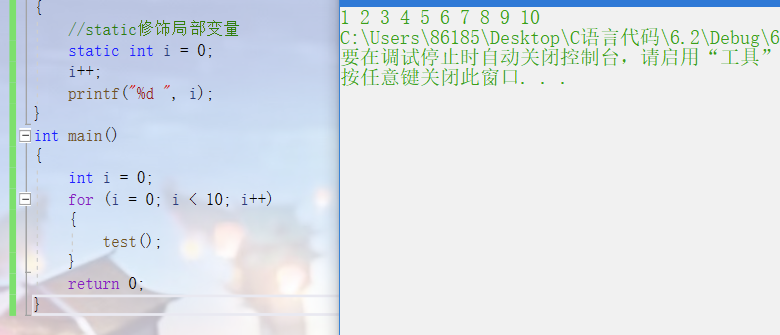test函数中的局部变量i在test函数运行结束后并没有被销毁，每次调用test函数都会使i自增。

``````#include <stdio.h>
void test()
{

//static修饰局部变量
int i = 0;
i++;
printf("%d ", i);
}
int main()
{

int i = 0;
for (i = 0; i < 10; i++)
{

test();
}
return 0;
}
``````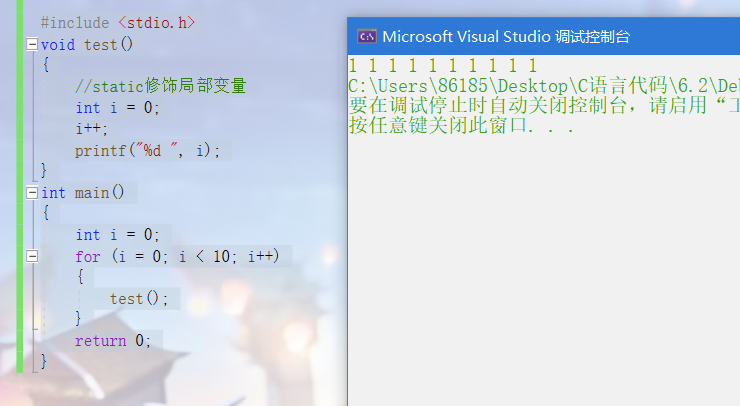• static 修饰全局变量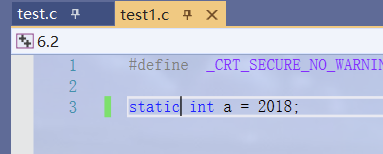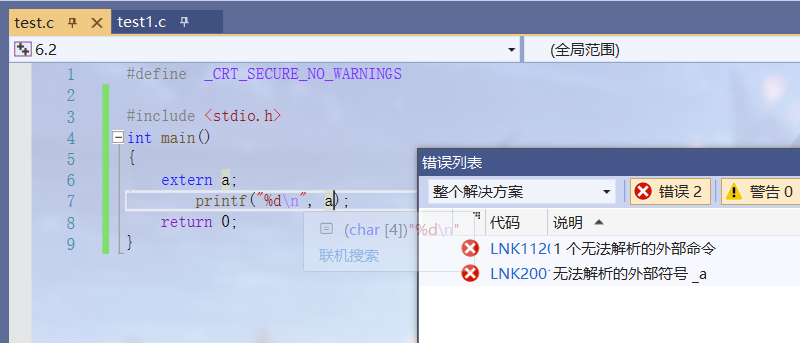• static修饰函数

# define定义常量和宏

#defne 宏名（被替换的内容）（要替换的内容）

``````//define定义标识符常量
#define MAX 1000//在程序中，只要遇到MAX，都会被替换为1000
//define定义宏
#include <stdio.h>
int main()
{

printf("%d\n", MAX);
printf("sum = %d\n", sum);
printf("sum = %d\n", sum);
return 0;
}
``````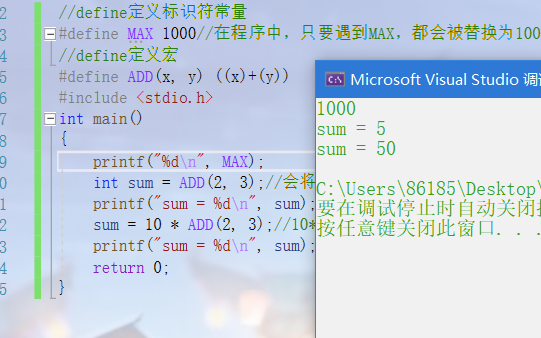# 指针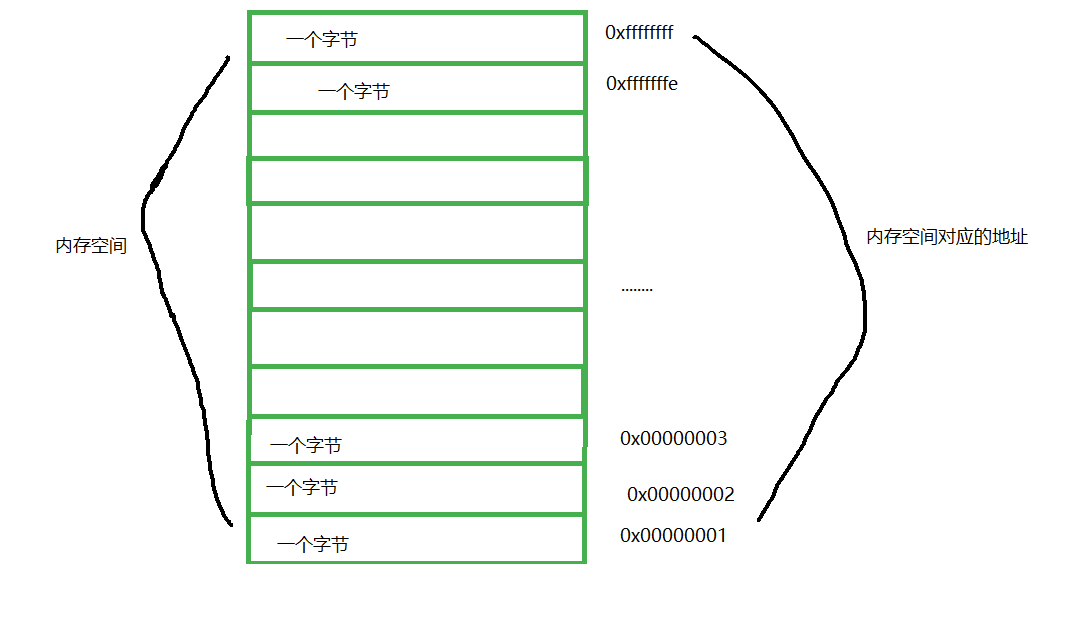``````int num = 10;
int *p;//p为一个整形指针变量
p = &num;
``````

&操作符可以将num的地址取出，然后赋值给指针p。

``````#include <stdio.h>
int main()
{

int num = 10;
int *p = &num;
*p = 20;//*是解引用操作符，*p是通过p找到p指向的对象，即num
return 0;
}
``````

*是解引用操作符，*p是通过p找到p指向的对象，即num

p也是一个指针变量，它也有地址。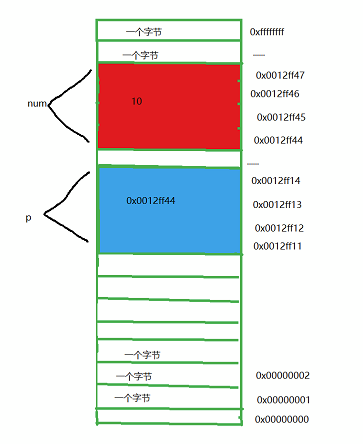## 指针的大小

``````#include <stdio.h>
int main()
{

printf("%d\n", sizeof(char *));//4
printf("%d\n", sizeof(short *));//4
printf("%d\n", sizeof(int *));//4
printf("%d\n", sizeof(double *));//4
return 0;
}
``````

# 结构体

``````struct Stu
{

char name;//名字
int age;      //年龄
char sex;  //性别
char id； //学号
};
int main()
{

struct Stu s = {
"张三"， 20， "男"， "20180101"};//创建一个学生s，并对其进行初始化赋值
//.为结构成员访问操作符，能够访问结构体的成员
printf("name = %s age = %d sex = %s id = %s\n", s.name, s.age, s.sex, s.id);
//创建一个结构体指针，用来存放s的地址
struct Stu *ps = &s;
//->操作符可以通过指针来访问到结构体的具体成员
printf("name = %s age = %d sex = %s id = %s\n", ps->name, ps->age, ps->sex, ps- >id);
}
``````

我有话说: